# Negative and Positive Number LineHello, and welcome to this video about the number line! In this video, we will explore how to read number lines, draw and plot points on a number line, and add and subtract with a number line. Let’s get started!

We will use a number line to represent numbers in counting order. The number to the left is always smaller than the number to its right. To draw a number line, we first start by drawing a straight line and then we put arrows at the ends. The arrows represent the fact that the number line goes on forever in both directions. When dividing the number line with tick marks, it is important to place the tick marks equally spaced apart. This shows that the distance from any two numbers is the same. For example, the distance from 1 to 2 is the same as the distance from 2 to 3.

The most important number on a number line is 0 because every other number is based off of how far away it is from 0. Numbers to the left of 0 are negative, and numbers to the right of 0 are positive. Number lines continue infinitely in either direction, so the number line you see is always only part of the whole number line. Because of this, even though 0 is the most important number, it might not show up on your number line. For example, your number line might look like this if you are trying to plot the grade you got on your test.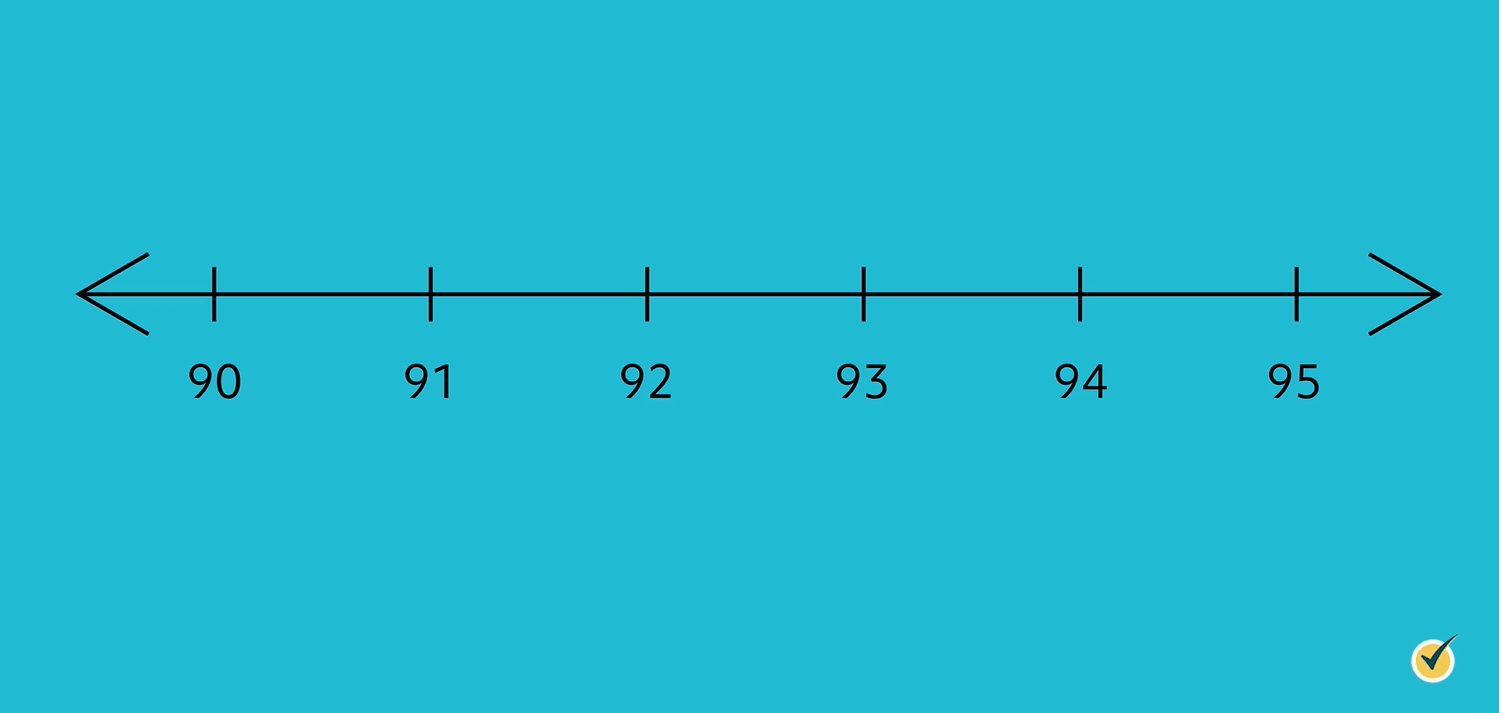Number lines can be used to represent a specific number. In the example we just mentioned, we can use the number line to represent a test grade of 94 by putting a dot above the number, like this: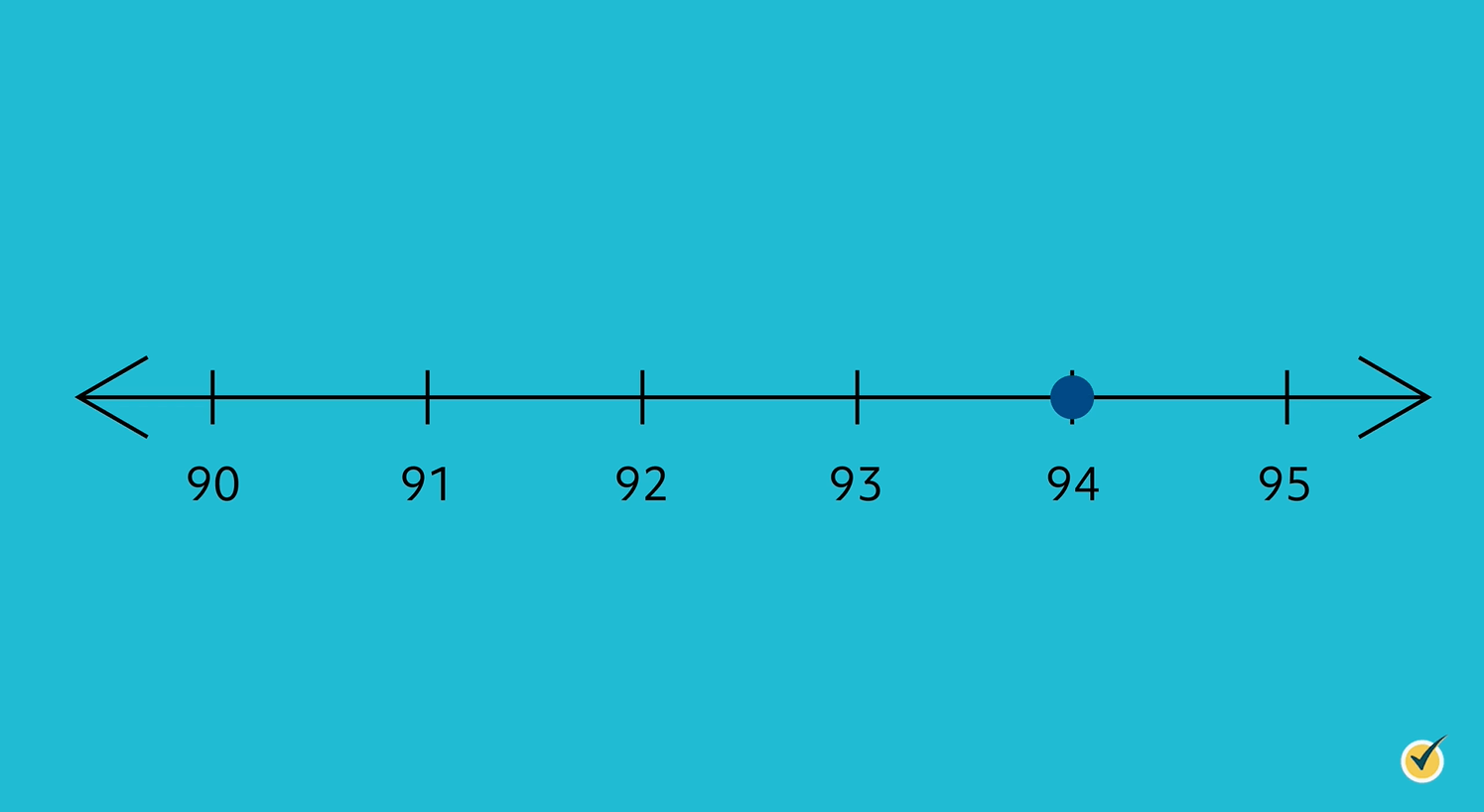Plotting a negative number would happen the same way. Simply put a dot over the number you are wanting to represent. The number line here shows the number -5.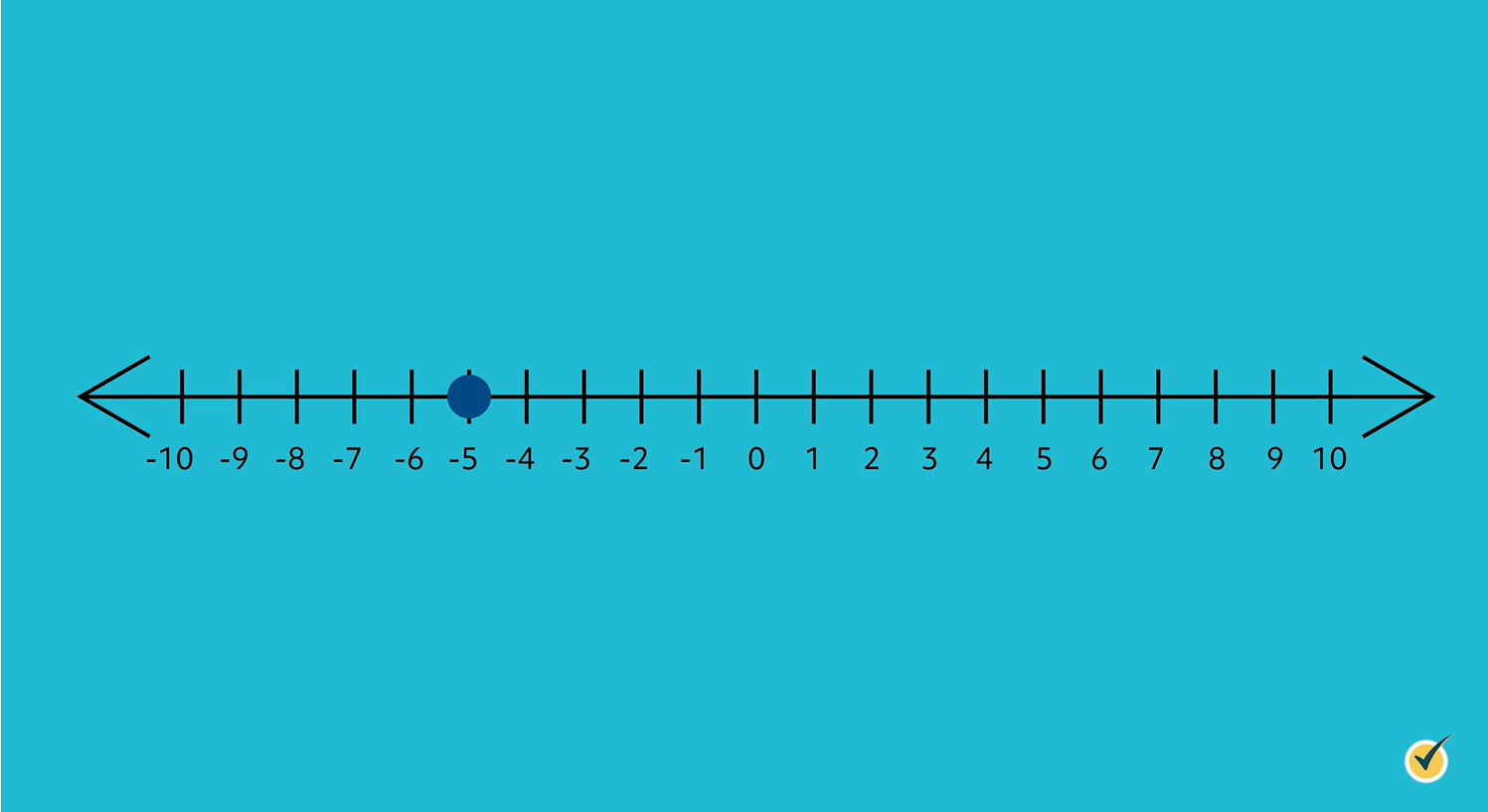We can also use a number line to help us add and subtract numbers. The movement to the right is for addition.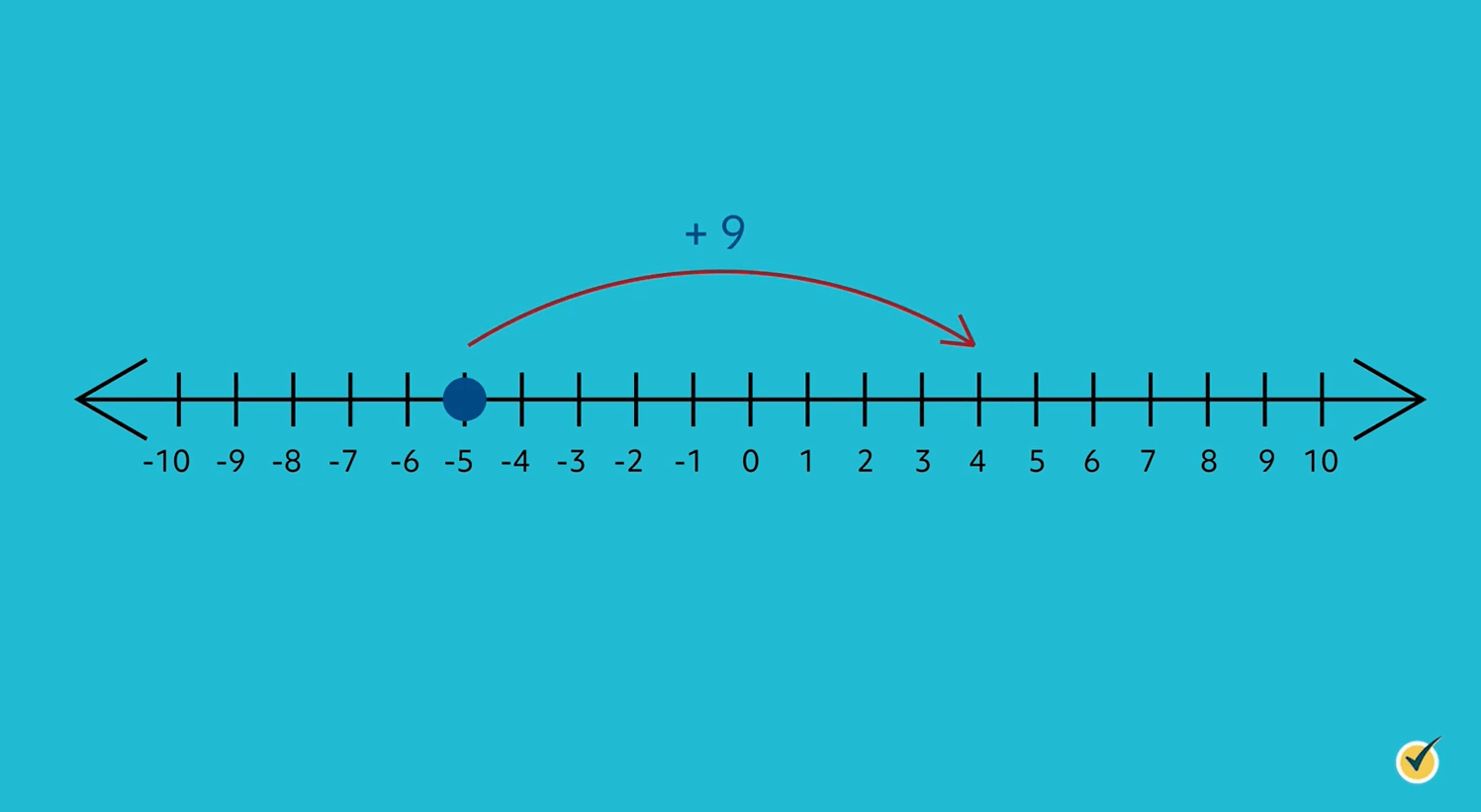The movement to the left is for subtraction.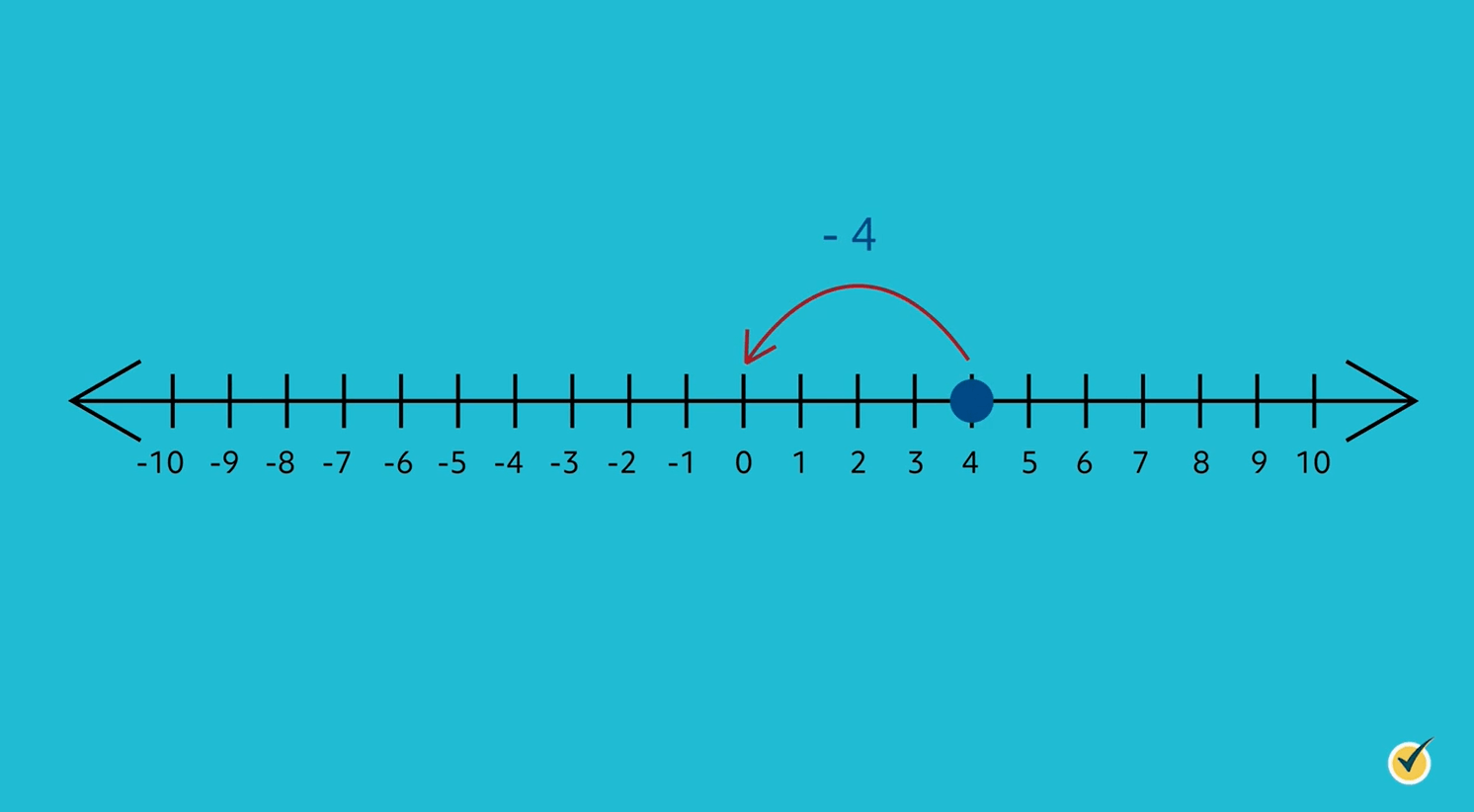To use a number line for adding and subtracting, we start by plotting a point at our first number. Then we move right or left (depending on the operation) the number of spaces matching the second number.

Let’s practice by adding -7+5. We will start at -7, and since the operation is addition, we will move to the right 5 spaces. As you can see, we land on -2. Therefore, -7+5=-2.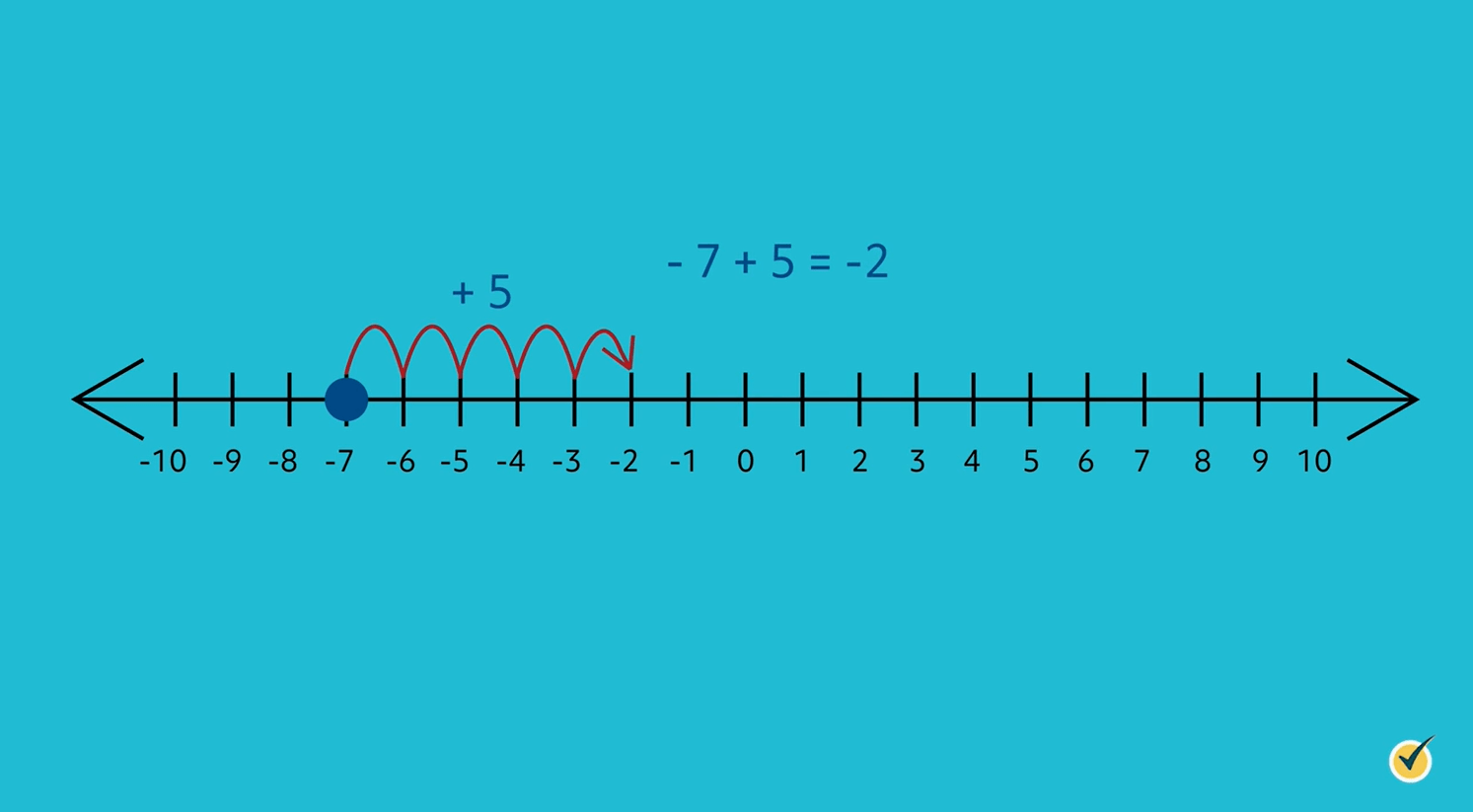Now let’s try subtracting 9-4. We will start at the number 9 on the number line. Since the operation is subtraction, this time we’re going to move to the left. We move left 4 spaces, which lands us on 5. Therefore, 9-4=5.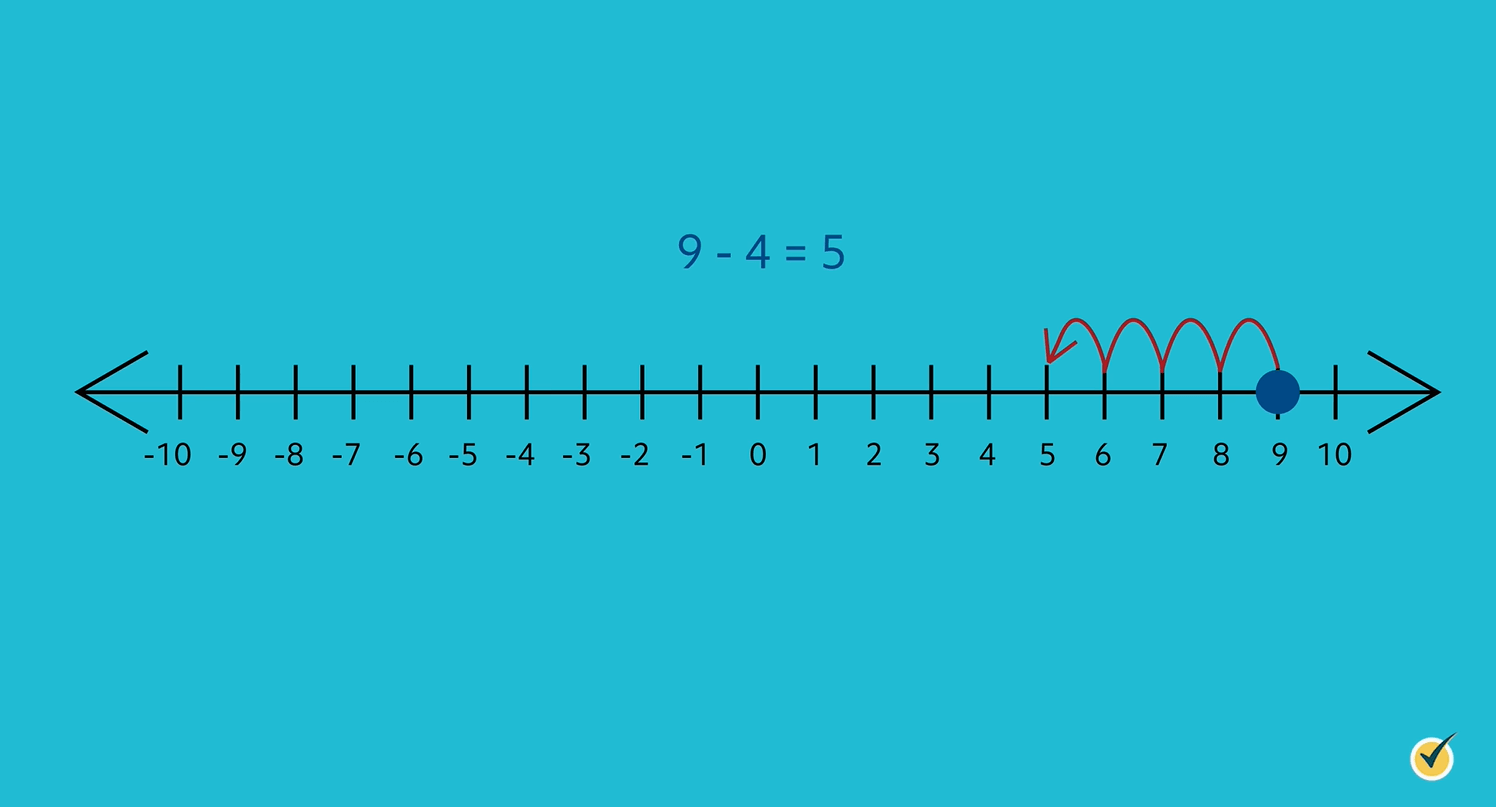I hope that this video on number lines was helpful. Thanks for watching, and happy studying!

## Practice Questions

Question #1:

Which scenario matches the number line below?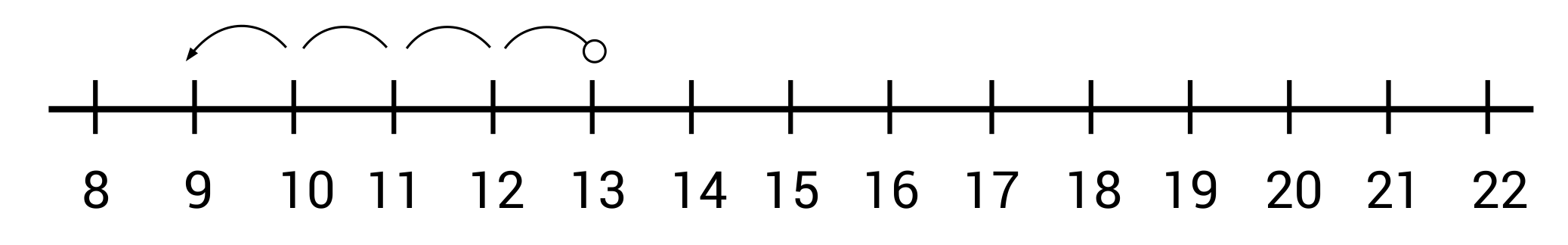Beth is selling bracelets at an art fair. She starts her day with nine bracelets, and then she sells four of them. Now she only has five more bracelets to sell.

Tony goes to the grocery store with nine dollars. He spends thirteen dollars for cereal and milk, and now he has four dollars left.

Tanya has thirteen dog treats in her pocket. She gives her puppy four of the treats, so now she has nine treats left.

John earns thirteen dollars mowing the neighbor’s lawn. On his way home, he spends nine dollars on a snack. Now John only has four dollars left.

Tanya starts with thirteen treats in her pocket, and then gives her puppy four of them. This is represented on the number line as four jumps back to the left from the number thirteen, landing on the number nine. In other words, $$13-4=9$$.

Question #2:

Which math sentence matches the number line below? Note that the original starting point is at zero.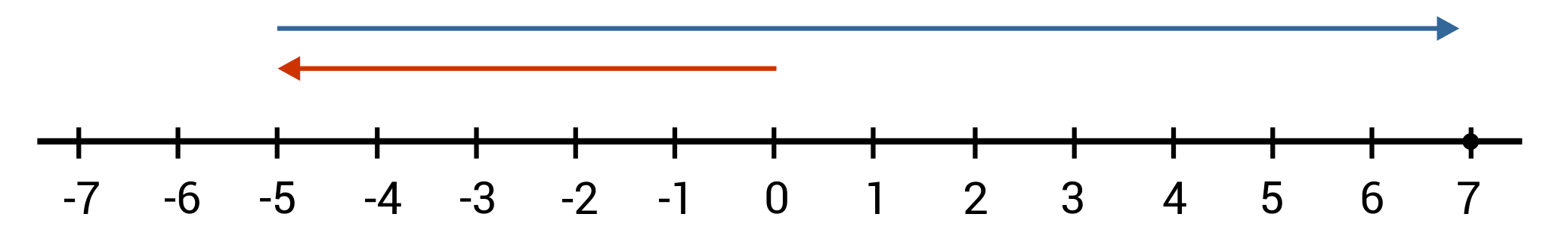$$0+12-5=7$$
$$0-7+12=5$$
$$0+5-12=7$$
$$0-5+12=7$$

Starting at 0, we move left (back) five jumps and land on -5. From -5, we then move to the right 12 jumps, landing on 7. Zero minus five, plus twelve, equals seven, which matches the number sentence $$0-5+12=7$$.

Question #3:

Which number line correctly plots the point 1.5?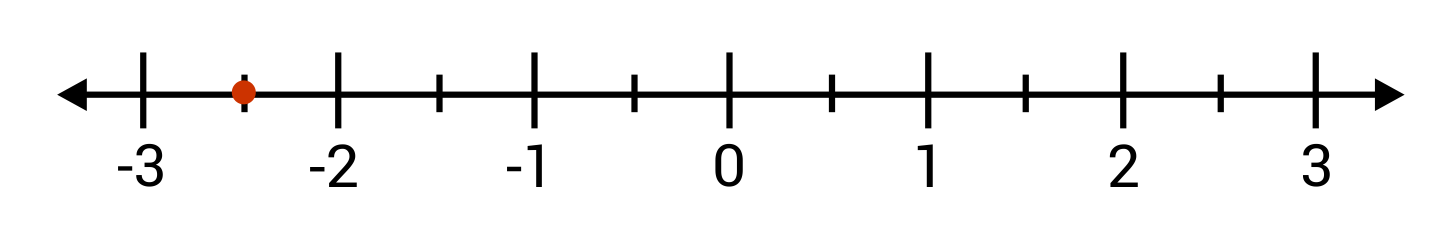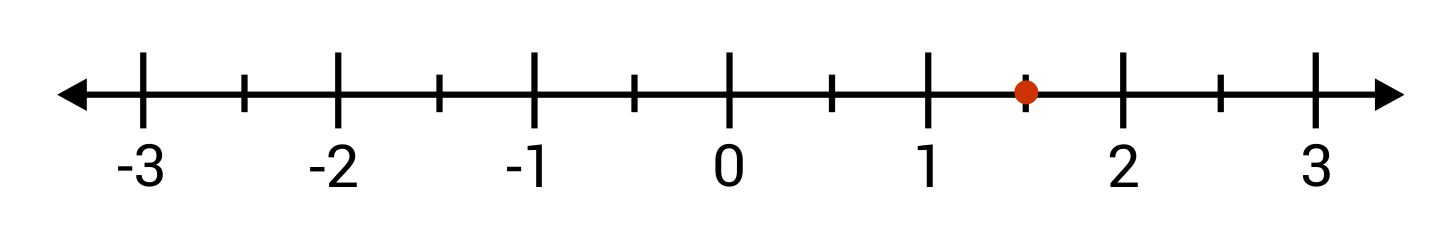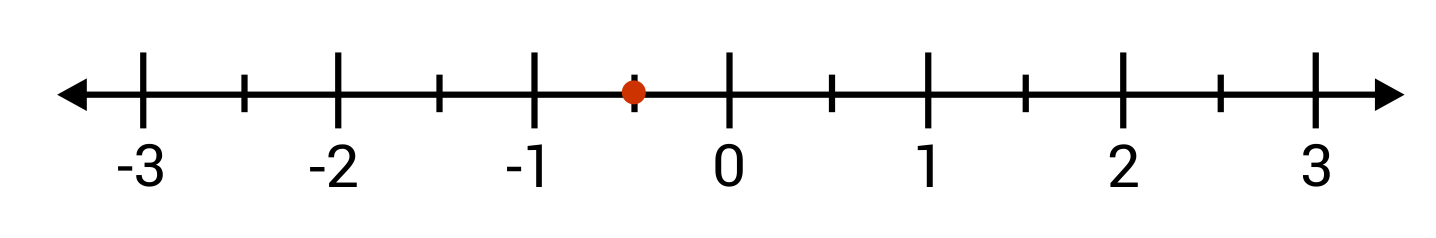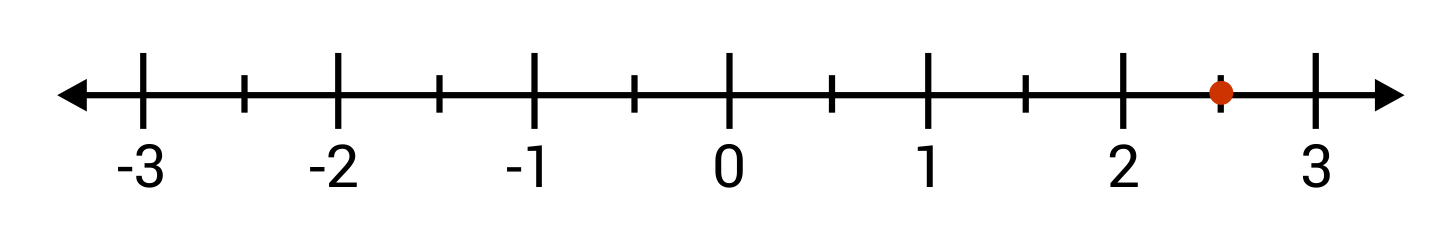Number line B shows the point 1.5 correctly plotted between 1 and 2.

Question #4:

Joey paints 11 picture frames blue, and 8 picture frames green. He paints a total of 19 picture frames. A number line is used to represent the total number of frames painted. Identify the error in the number line below.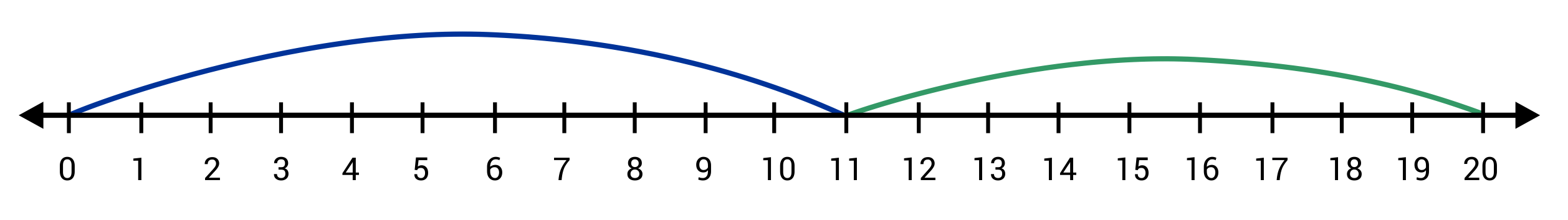The green line is incorrect. It shows a jump of 9, but it should show a jump of 8.

The blue line is incorrect. It should show a jump of 12, not 11.

Both lines are incorrect.

The green line is incorrect. It should show a jump of 13, not 9.

Joey painted 11 blue frames, so the blue line is correct in showing a jump of 11. He painted 8 frames green, so the green line should show a jump of 8, from 11 to 19. However, the green line is incorrect. The green line shows a jump of 9, landing on 20.

Question #5:

Fill in the missing value so that the number line matches the number sentence:
$$3+\text{___}=8$$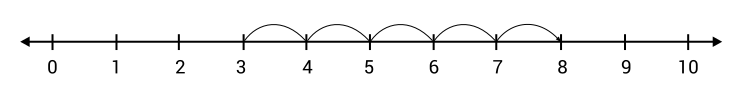4

5

6

7

The number line shows a starting point of 3, and then 5 jumps to the right, eventually landing on the number 8. The number line matches the number sentence $$3+5=8$$, so the missing value is 5.